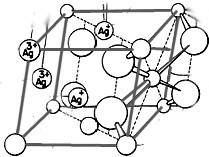rexresearch.com

Marvin ANTELMAN
TetraSilver Tetroxide ( TSTO )

Introductory Brochure
Reports

Patents
Preparation & Properties
ProductsTools:
https://www.bocsci.com/molarity-calculator.html     Molarity Calculator -- Calculate Mass Required for Molar Solution
https://www.bocsci.com/molecular-weight-calculator.html     Molecular Weight Calculator -- Enter the chemical formula of a compound to calculate its molar mass and elemental composition
https://www.bocsci.com/solution-dilution-calculator.html     Solution Dilution Calculator -- Dilute Solution of Known Molarity
https://www.rapidtables.com/math/number/PPM.html     PPM - parts per million
https://www.physiologyweb.com/calculators/dilution_calculator_ppb_ppm_ppt_pph.html     Dilution Calculator - ppb, ppm, ppt, pph